# Classification of Discontinuity JEE Notes | EduRev

## JEE : Classification of Discontinuity JEE Notes | EduRev

The document Classification of Discontinuity JEE Notes | EduRev is a part of the JEE Course Mathematics (Maths) Class 12.
All you need of JEE at this link: JEE

B. Classification of Discontinuity

Definition :– Let a function f be defined in the neighbourhood of a point c, except perhaps at c itself.

Also let both one–sided limits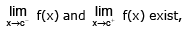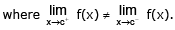Then the point c is called a discontinuity of the first kind in the function f(x).

In more complicated case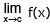may not exist because

one or both one-sided limits do not exist. Such condition is called a discontinuity of the second kind.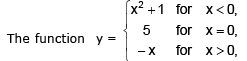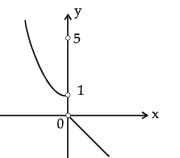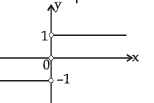has a discontinuity of the first kind at x = 0

The function y = |x| /x is defined for all x ∈ R, x ≠ 0;

but at x = 0 it has a discontinuity of the first kind.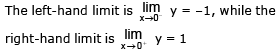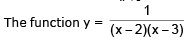has no limits (neither one-sided nor  two-sided) at x = 2 and x = 3 since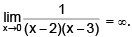Therefore x = 2 and x = 3 are discontinuities of the second kind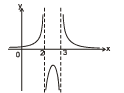The function y = ln |x| at the point x = 0 has the limits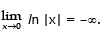Consequently,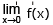(and also the one-sided limits) do not exist; x = 0 is a discontinuity of the second kind.

It is not true that discontinuities of the second kind only arise whenThe situation is more complicated.
Thus, the function y = sin (1/x), has no one-sided limits for x → 0 and x →  0+, and does not tend to infinity as x →  0  There is no limit as x →  0 since the values of the function sin (1/x) do not approach a certain number, but repeat an infinite number of times within the interval from –1 to 1 as x→ 0.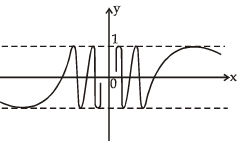Removable & Irremovable Discontinuity

(a) In case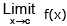exists but is  not equal to f(c) then the function is said to have a removable discontinuity. In this case we can  redefine the function such that= f(c) & make it continuous at x = c.

Removable Type Of Discontinuity Can Be Further Classified As :

(i) Missing  Point  Discontinuity   :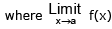exists finitely but f(a)  is not  defined .

e.g.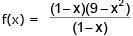has  a  missing  point discontinuity at  x = 1

(ii) Isolated  Point  Discontinuity :exists & f(a) also exists but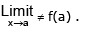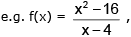x ≠ 4 &  f (4) = 9 has a break  at x = 4.

(b)  In  case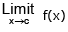does not exist then  it is not  possible to make the function continuous by redefining it . Such  discontinuities  are known  as non - removable discontinuity.

Irremovable Type Of Discontinuity Can Be Further Classified As :

(i) Finite discontinuity :   e.g. f(x) = x - [x] at all integral  x.

(ii) Infinite discontinuity :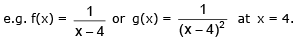(iii) Oscillatory discontinuity :     e.g. f(x) = sin1/x at x = 0

In all these cases the value of f(a) of the function at x= a (point of discontinuity) may or may not exist butdoes not  exist.

Remark :

(i) In case of finite discontinuity the non-negative difference between the value of the RHL at x = c & LHL at x = c is called The Jump Of Discontinuity . A function having a finite number of jumps in a given interval I is called a Piece-wise Continuous or Sectionally Continuous function in this interval.

(ii) All Polynomials, Trigonometrical functions, Exponential & Logarithmic functions are continuous in their domains.

(iii) Point  functions are to be treated as discontinuous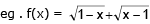is not continuous at  x = 1.

(iv) If  f is  continuous at  x = c &  g is continuous  at x = f(c)  then  the  composite g[f(x)] is continuous at x = c.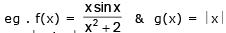are continuous at x = 0 , hence the  composite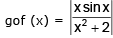will  also  be  continuous  at x = 0.

Offer running on EduRev: Apply code STAYHOME200 to get INR 200 off on our premium plan EduRev Infinity!

## Mathematics (Maths) Class 12

209 videos|222 docs|124 tests

,

,

,

,

,

,

,

,

,

,

,

,

,

,

,

,

,

,

,

,

,

;